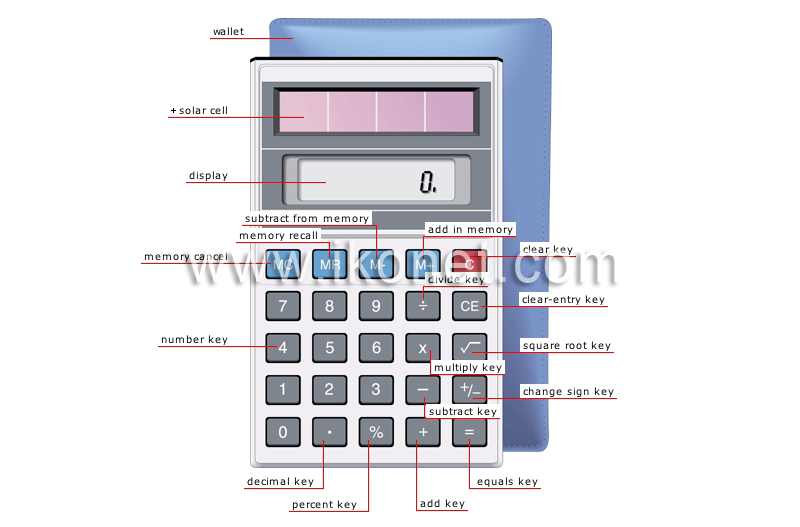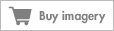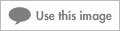Look up an image:

#### pocket calculatorSmall self-powered electronic instrument used to automatically make numerical calculations.See pocket calculator in : french | spanish

#### equals keyKey used to display the results of operations carried out.

#### change sign keyKey used to change the plus or minus sign of the displayed number.

#### subtract from memoryKey used to erase the displayed number from memory.

#### add in memoryKey used to save the displayed number in memory.

#### clear keyKey used to return the pocket calculator to zero.

#### divide keyKey used to calculate the quotient of two numbers.

#### clear-entry keyKey used to erase the last number entered.

#### square root keyKey used to derive the square root of a number; this is the number that is multiplied by itself to give the basic number.

#### multiply keyKey used to calculate the product of two numbers.

#### decimal keyKey used to insert a decimal symbol to separate the whole and fraction parts of a number.

#### subtract keyKey used to calculate the difference between two numbers.

#### number keyKey used to enter a number.

#### memory cancelButton that erases data stored in memory.

#### memory recallButton that displays data stored in memory.

#### displayLiquid crystal display that shows the last number entered or the result of operations carried out.

#### solar cellDevice that converts sunlight into electric current to power a pocket calculator.

#### percent keyKey used to obtain the decimal form of a displayed number by dividing it by 100; it is used mainly to calculate percentages.

#### add keyKey used to add two numbers.